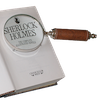#### You may also like### Writing Digits

Lee was writing all the counting numbers from 1 to 20. She stopped for a rest after writing seventeen digits. What was the last number she wrote?### Number Detective

Follow the clues to find the mystery number.### Six Is the Sum

What do the digits in the number fifteen add up to? How many other numbers have digits with the same total but no zeros?

##### Age 5 to 11Challenge Level

Here's a maths trick to try:

1. Think of one of the numbers from 1 to 9.

What do you notice?

Try the trick again with a new starting number from 1 to 9. What do you notice now?

Will this always happen if you start with a number from 1 to 9?

Jonas tried the trick with a few different numbers. He said:

I think you will always get back to your original number, because adding 9 is the same as adding 10 and subtracting 1.

Do you agree with Jonas? Can you help him explain what is happening?

Can you explain the trick in a different way?

### Why do this problem?

'Tricks' tap into children's natural curiosity and can provide the motivation for exploring the underlying mathematics in order to unpick how they are done. This trick provides an engaging context in which to explore place value and in particular 'adding nine' as 'adding ten and subtracting one'.

### Possible approach

This problem featured in the NRICH Primary webinar in June 2022.

With the whole class, practise adding 9 to a variety of one-digit numbers. Introduce the trick and discuss what 'digit addition' means. As a class, choose a number between 1 and 9 (inclusive) and work through the steps together on the board. What has happened? Is it magic?

In pairs, ask pupils to choose numbers from 1 to 9, and work through the steps with each number. Working in pairs will allow pupils to check and correct each other's addition, ensuring that the 'trick' always gives back the starting number. You may wish to suggest that particular pairs investigate certain starting numbers so that as a class you have covered all the numbers from 1 to 9.

Once each pair has tried a few numbers, bring the class back together. Ask if anyone has their own ideas about what is happening, and then show the class Jonas' explanation. What does he mean? Why are 10s and 1s interesting?

Choose a starting number and represent that number on the board, either using counters in a place value grid or using 1s counters to represent the number. Work out the answer after 9 has been added to it. Represent this number on the board underneath the first number, either using counters in the place value grid or using a 10s counter and some 1s counters. What can pupils say about the number of counters? What does the digit addition have to do with the number of counters? (When we add the digits, we add the tens and ones digits together as if all the counters had the same value.)

A different approach would be to write some of the numbers on the board and show the different steps of the trick, e.g.:

3 + 9 = 12, 1 + 2 = 3
4 + 9 = 13, 1 + 3 = 4
5 + 9 = 14, 1 + 4 = 5

Ask the children what they notice. Can they see any patterns? What happens when the starting number gets bigger by one?